# Texas Go Math Grade 1 Lesson 9.2 Answer Key Count Collections of Coins

Refer to our Texas Go Math Grade 1 Answer Key Pdf to score good marks in the exams. Test yourself by practicing the problems from Texas Go Math Grade 1 Lesson 9.2 Answer Key Count Collections of Coins.

## Texas Go Math Grade 1 Lesson 9.2 Answer Key Count Collections of Coins

Essential Question
How can you count a group of pennies, nickels, and dimes?
We can count by Cents

Explore
Use coins to show the problem. Draw and label the coins.

For The Teacher

• Juan has some pennies, nickels, and dimes. He chooses one kind of coin to show 20 cents. How can Juan show 20 cents?
Explanation:
1 dime = 10¢
2 x 10 = 20 ¢
So, he choose dime.

Math Talk
What coins have the same value as 10 pennies? Explain.
Explanation:
A Dime has same value of 10 pennies

Model and Draw

Count to find the total value.

Why would you start dimes?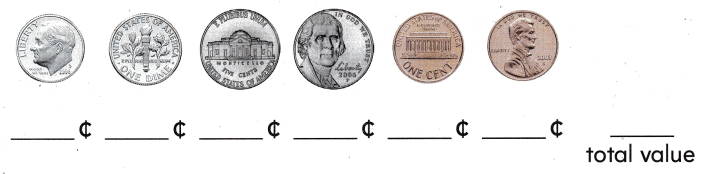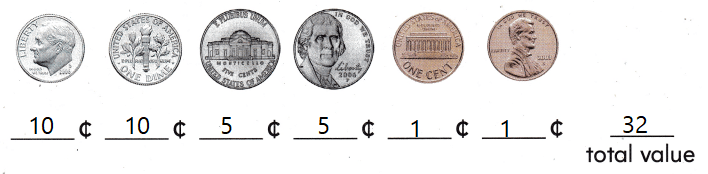Explanation:
2 dimes = 20¢
2 Nickel = 10¢
2 pennies = 2¢

Share and Show

Count. Write the total value.

Question 1.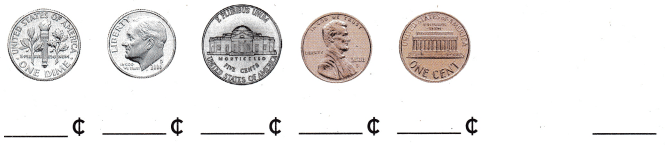Explanation:
1 dime = 10¢ = 20
1 nickel = 5¢ = 5
1 penny = 1¢ = 2
10 + 5 + 2 = 27¢

Question 2.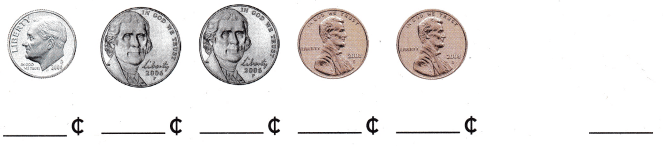Explanation:
1 dime = 10¢ = 10
1 nickel = 5¢ = 10
1 penny = 1¢ = 2
10 + 10 + 2 = 22¢

Question 3.Explanation:
1 dime = 10¢ = 20
1 nickel = 5¢ = 15
1 penny = 1¢ = 1
20 + 15 + 1= 36¢

Problem Solving

Count by ones, fives, or tens. Write the total value.

Question 4.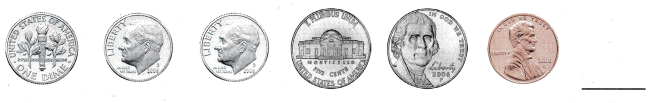Explanation:
1 dime = 10¢ = 30
1 nickel = 5¢ = 10
1 penny = 1¢ = 1
30 + 10 + 1= 41¢

Question 5.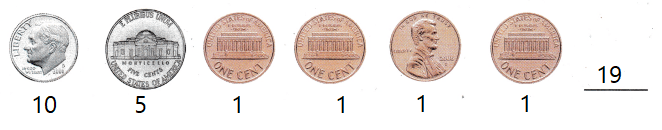Explanation:
1 dime = 10¢ = 10
1 nickel = 5¢ = 5
1 penny = 1¢ = 4
10 + 5 + 4 = 19¢

Question 6.Explanation:
1 dime = 10¢ = 0
1 nickel = 5¢ = 15
1 penny = 1¢ = 3
15 + 3 = 18¢

Question 7.
Karl has 4 nickels and 3 pennies. Show the same amount with fewer coins.1 Nickel = 5 cents
1 penny = 1 cent
Explanation:
4 x 5 = 20
3 x 1 = 3
20 + 3 = 23

Question 8.
Multi-Step Elsie has 2 dimes. Tyler has four coins with the same value. Draw the coins Tyler has.

Describe how a dime and a nickel are related.
1 dime = 10 cents
1 nickel = 5 cents
Explanation:
Elsie has 2 dimes. Tyler has four coins with the same value.
2 x 10 = 20
4 x 5 = 20
20 = 20
Tyler has Nickels.

Use coins. Choose the correct answer.

Question 9.
Apply Matt wants to buy the magnet. Which coins does he need?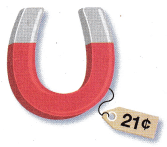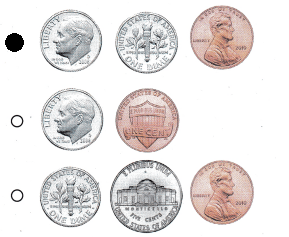Explanation:
2 x 10 = 20 cents
1 penny = 1 cent
20 + 1 = 21Cents

Question 1o.
Multi-Step Meg has a dime. Yuri has 2 coins. Each has the same amount of money. What coins does Yuri have?
(A) 2 pennies
(B) 2 dimes
(C) 2 nickels
Explanation:
1 dime = 10 cents
1 nickel = 5 cents
Meg has a dime. Yuri has 2 coins.
2 x 5 = 10
So, Yuri has nickels.

Question 11.
Texas Test Prep What is the total value?(A) 40¢
(B) 31¢
(C) 26¢
Explanation:
2 x 10 = 20
1 x 5 = 5
1 x 1 = 1
20 + 5 + 1 = 26¢

Take Home Activity

• Have your child count to identify the total value of groups of pennies, nickels, and/or dimes, up to 50 cents.

### Texas Go Math Grade 1 Lesson 9.2 Homework and Practice Answer Key

Count. Write the total value.

Question 1.Explanation:
1 dime = 10 cents 1 x 10 = 10
1 nickel =5 cents 1 x 5 = 5
1 penny = 1 x 3 = 3 cent
10 + 5 + 3 = 18

Question 2.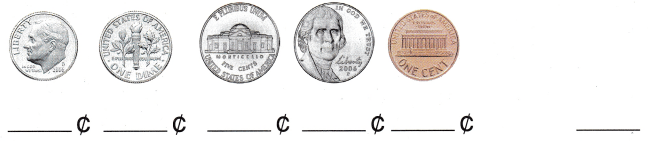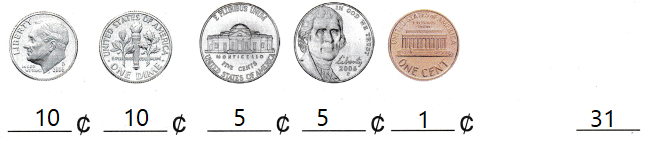Explanation:
1 dime = 10 cents 2 x 10 = 20
1 nickel =5 cents 2 x 5 = 10
1 penny = 1 cent
20 + 10 + 1 = 31

Question 3.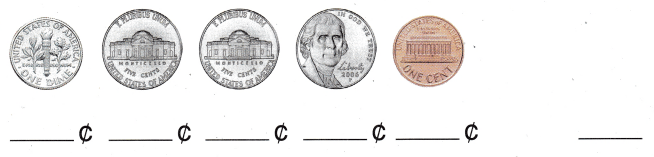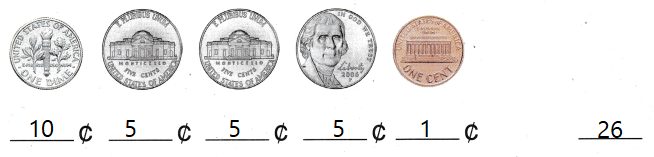Explanation:
1 dime = 10 cents 1 x 10 = 10
1 nickel =5 cents 3 x 5 = 15
1 penny = 1 cent
10 + 15 + 1 = 26

Problem Solving

Draw and label coins to solve.

Question 4.
Ellie has 5 nickels and 6 pennies. Show the same amount with fewer coins.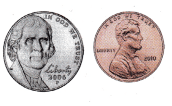Explanation:
1 Nickel = 5 cents
1 penny = 1 cent

Texas test prep

Lesson Check

Question 5.
Frank finds these coins in his book bag. What is the total value of the coins?(A) 56¢
(B) 26¢
(C) 46¢
Explanation:
1 dime = 10 cents 4 x 10 = 40
1 nickel =5 cents 1 x 5 = 5
1 penny = 1 cent 1 x 1 = 1
40 + 5 + 1 = 46¢

Question 6.
Mrs. Walters wants to buy a bottle of glue. Which coins does she need?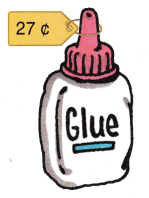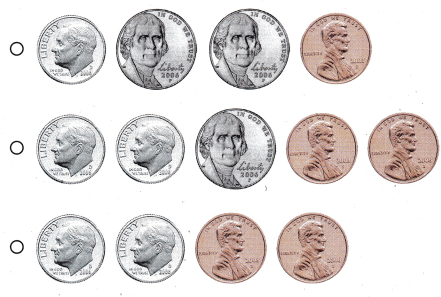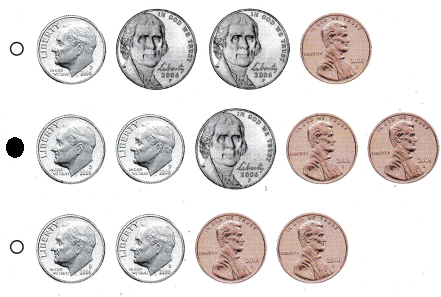Explanation:
1 dime = 10 cents 2 x 10 = 20
1 nickel =5 cents 1 x 5 = 5
1 penny = 1 cent 2x 1 = 2
20 + 5 + 2 = 27¢

Question 7.
Multi-Step Isabelle has 2 coins. Her mom gives her 2 more. Now Isabelle has 20¢. What coins does Isabelle
have now?
(A) 2 pennies
(B) 2 dimes
(C) 4 nickels